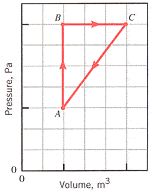# How much heat is converted into work?

1) Five thousand joules of heat is put into a Carnot engine whose hot and cold reservoirs have temperatures of 500 and 250 K, respectively. How much heat is converted into work?

here's what i did.....
i know that.... QC / QH = TC / TH
W = 5000, Tc = 250, Th = 500, Qh = ?, Qc = ?

first I found Qh , which = (eCarnot = W/Qh) ----- and solve for Qh and get (Qh = W/eCarnot) ------eCarnot also equals [1-(Tc/Th)] = [1-( 250 / 500 ) ] = 0.5
so Qh = W / [1-(Tc/Th) = 5000 / 0.5 = 10000
after I got Qh I used the equation (Qc = Qh - W) to solve for Qc and this should be the answer...
Qc = Qh - W = 10000-5000 = 5000 , so Qc = 5000 ...but it still wrong :surprised :yuck:2) The pressure and volume of a gas are changed along a path ABCA in the figure. The vertical divisions on the graph represent 4.5 105 Pa, and the horizontal divisions represent 4.0 10-3 m3. Determine the work done (including algebraic sign) in each segment of the path.a ) A to B = ? J
b ) B to C = ? J
c ) C to A = ? J

This question..i know that W= P ( Vf-Vi )
but i still cant locate the dots on the graph!!Hootenanny
Staff Emeritus
Gold Member
For question one the maximum efficencey of a carnot engine is given by;

$$1 - \frac{T_{C}}{T_{H}}$$

This will give you a fraction. This in the fraction of the energy which can be used (i.e. work done). So all you need to do is multiply the input energy ($Q_{H}$) by this fractional efficency.

-HootAndrew Mason
Homework Helper
iceT said:
1) Five thousand joules of heat is put into a Carnot engine whose hot and cold reservoirs have temperatures of 500 and 250 K, respectively. How much heat is converted into work?
The 5000 J. of heat represents Qh not W.

W = .5 Qh = 2500 J.

So only 2500 J of the 5000 is converted into work.

2) The pressure and volume of a gas are changed along a path ABCA in the figure. The vertical divisions on the graph represent 4.5 105 Pa, and the horizontal divisions represent 4.0 10-3 m3. Determine the work done (including algebraic sign) in each segment of the path.

a ) A to B = ? J
b ) B to C = ? J
c ) C to A = ? J

This question..i know that W= P ( Vf-Vi )
but i still cant locate the dots on the graph!!The work done is the area under the graph between those points.

AM